title: Bayesian Factor Analysis Regression in Python with PyMC3

## tags: PyMC3, Bayesian, Python¶

Wikipedia defines factor analysis as

a statistical method used to describe variability among observed, correlated variables in terms of a potentially lower number of unobserved variables called factors.

Factor analysis is used frequently in fields such as psychometrics, political science, and many others to identify latent (unmeasurable) traits that influence the observable behavior of people and systems.

Mathematically, Machine Learning: A Probabilistic Perspective (my favorite ML reference) describes factor analysis as "a low rank parametrization of [a multivariate normal distribution]" and as "a way of specifying a joint density model on [the vector] $\mathbf{x}$" using a small number of parameters.

This post will show how to add a richer covariance structure to the analysis of a simulated multivariate regression problem using factor analysis in Python with PyMC3. As we will see, specifying this model is somewhat tricky due to identifiability issues with naive model specifications.

## Simulated data¶

We begin by simulating the data that we will subsequently analyze. First we make the necessary python imports and do some light housekeeping.

In :
%matplotlib inline

In :
from warnings import filterwarnings

In :
from aesara import tensor as at
import arviz as az
from matplotlib import pyplot as plt
import numpy as np
import pymc3 as pm
import seaborn as sns

You are running the v4 development version of PyMC3 which currently still lacks key features. You probably want to use the stable v3 instead which you can either install via conda or find on the v3 GitHub branch: https://github.com/pymc-devs/pymc3/tree/v3

In :
filterwarnings('ignore', category=RuntimeWarning, module='arviz')
filterwarnings('ignore', category=UserWarning, module='arviz')
filterwarnings('ignore', category=UserWarning, module='pandas')

In :
plt.rcParams['figure.figsize'] = (8, 6)
sns.set(color_codes=True)


The observed predictors, $\mathbf{x}_i$, will be $5$-dimensional vectors with entries drawn i.i.d. from the standard normal distribution. We generate 100 such vectors.

In :
SEED = 123456789 # for reproducibility

rng = np.random.default_rng(SEED)

In :
N = 100
K = 5

In :
X = rng.normal(size=(N, K))


The observed targets, $\mathbf{y}_i$, will be 10-dimensional vectors.

The constant term for this model, $B_0$, is therefore a random vector in $\mathbb{R}^{10}$ whose entries are i.i.d. drawn from the uniform distribution on $[-3, 3]$.

In :
M = 10

In :
B0 = rng.uniform(-3., 3., size=M)


The component slopes form a $10 \times 5$ dimensional matrix. We generate a somewhat sparse matrix that has only (approximately) 50% of entries nonzero. These entries are also i.i.d. from the uniform distribution on $[-3, 3]$.

In :
B = np.zeros((M, K))
P_nonzero = 0.5

is_nonzero = np.random.binomial(1, P_nonzero, size=(M, K)) == 1
B[is_nonzero] = rng.uniform(-3., 3., size=is_nonzero.sum())


The observations before noise are

$$\mathbf{y}_i^{\text{noiseless}} = B_0 + B\ \mathbf{x}_i.$$
In :
Y_noiseless = B0 + X.dot(B.T)


So far this is a fairly standard simulation from a linear regression model. Things start to get more interesting when we introduce a non-diagonal correlation structure to the noise using latent factors. The noise added to the $j$-th component of the $i$-th sample is

$$\varepsilon_{i, j} = \mathbf{w}_j \cdot \mathbf{z}_i + \sigma_{i, j}$$

where $\mathbf{w}_1, \cdots \mathbf{w}_{10}$, and $\mathbf{z}_1, \ldots, \mathbf{z}_{100}$ are vectors in $\mathbb{R}^2$ whose entries are drawn i.i.d. from a standard normal distribution. Here two is the number of latent factors that govern the covariance structure. The uncorrelated noise is drawn i.i.d. from a normal distribution with variance $(0.25)^2$.

In :
F = 2

S_SCALE = 1.

In :
W = rng.normal(size=(M, F))
Z = rng.normal(size=(N, F))

S = rng.normal(scale=S_SCALE, size=(N, M))


We now add this noise to our noiseless "data" to produce the actual observations.

In :
Y = Y_noiseless + Z.dot(W.T) + S


Plotting the components of $\mathbf{x}_i$ against those of $\mathbf{y}_i$, we see (somewhat) sparse linear patterns emerge.

In :
fig, axes = plt.subplots(nrows=M, ncols=K,
sharex=True, sharey=True,
figsize=(20, 12))

for j, row_axes in enumerate(axes):
for i, ax in enumerate(row_axes):
ax.scatter(X[:, i], Y[:, j], alpha=0.5);

ax.set_xlabel(f"$x_{{{i + 1}}}$");
ax.set_ylabel(f"$y_{{{j + 1}}}$");

fig.tight_layout();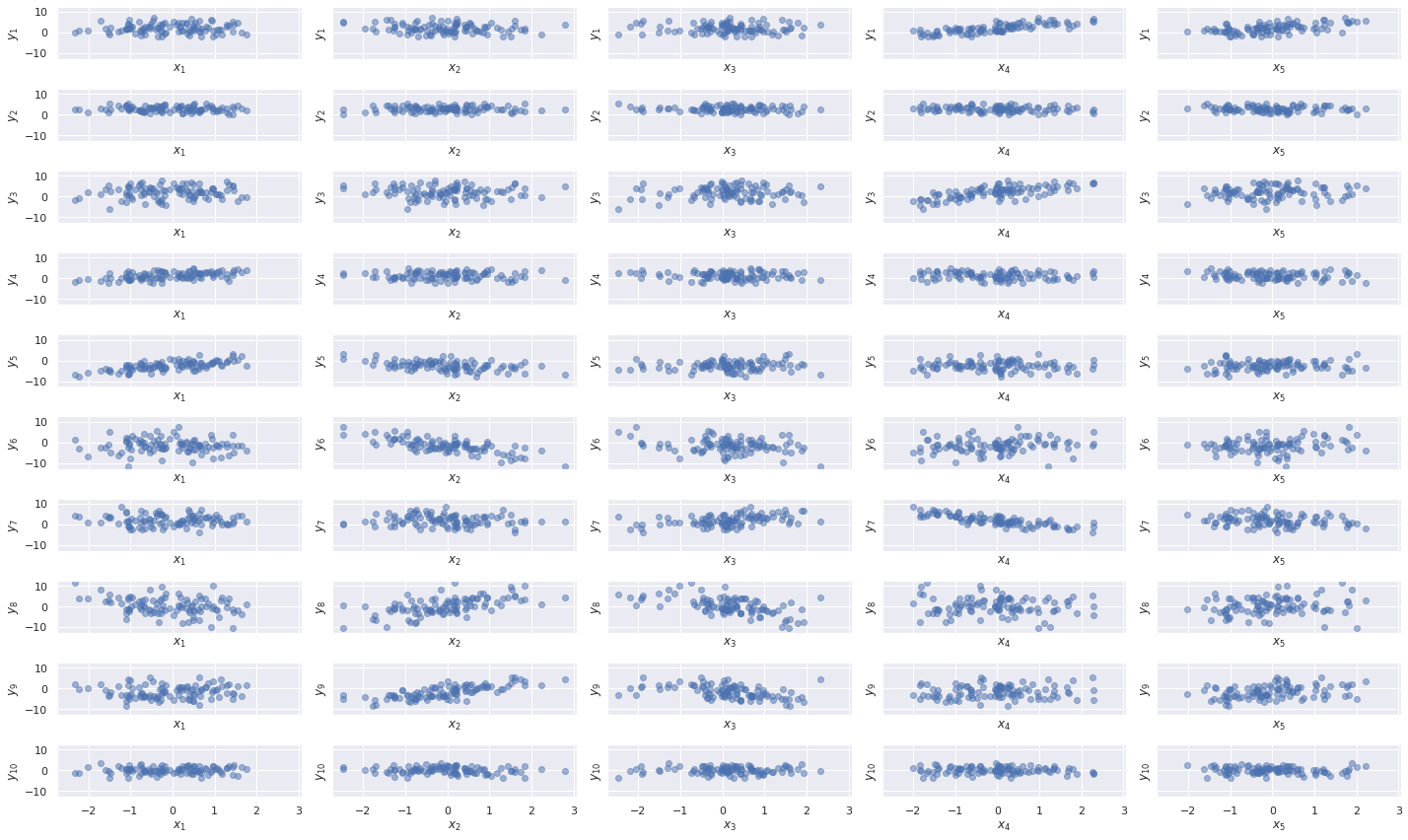## Modeling¶

We now begin building a series of models of this data using PyMC3.

### No latent factors¶

We start with a simple multivariate regression model that assumes diagonal covariance to see the impact of ignoring the latent factors on our model.

We place i.i.d. $N(0, 5^2)$ priors on the components of the intercepts ($\beta_0$) and the slopes ($\beta$).

In :
with pm.Model() as multi_model:
β0 = pm.Normal("β0", 0., 5., shape=M)
β = pm.Normal("β", 0., 5., shape=(M, K))


The noise scale gets the prior $\sigma \sim \text{Half}-N(2.5^2)$.

In :
with multi_model:
σ = pm.HalfNormal("σ", 2.5)


We now specify the likelihood of the observed data given the covariates.

In :
with multi_model:
μ = β0 + at.dot(X, β.T)
obs = pm.Normal("obs", μ, σ, observed=Y)


We now sample from the posterior distribution of these parameters.

In :
CORES = 4

SAMPLE_KWARGS = {
'cores': CORES,
'random_seed': [SEED + i for i in range(CORES)],
'return_inferencedata': True
}

In :
with multi_model:
multi_trace = pm.sample(**SAMPLE_KWARGS)

Auto-assigning NUTS sampler...
Multiprocess sampling (4 chains in 4 jobs)
NUTS: [β0, β, σ]

100.00% [8000/8000 00:50<00:00 Sampling 4 chains, 0 divergences]
Sampling 4 chains for 1_000 tune and 1_000 draw iterations (4_000 + 4_000 draws total) took 56 seconds.


Standard sampling diagnostics (energy plots, BFMI, and $\hat{R}$) show no cause for concern.

In :
def make_diagnostic_plots(trace, axes=None,
min_mult=0.995, max_mult=1.005,
var_names=None):
if axes is None:
fig, axes = plt.subplots(ncols=2,
sharex=False, sharey=False,
figsize=(16, 6))

az.plot_energy(trace, ax=axes)

rhat = az.rhat(trace, var_names=var_names).max()
axes.barh(np.arange(len(rhat.variables)), rhat.to_array(),
tick_label=list(rhat.variables.keys()))
axes.axvline(1, c='k', ls='--')

axes.set_xlim(
min_mult * min(rhat.min().to_array().min(), 1),
max_mult * max(rhat.max().to_array().max(), 1)
)
axes.set_xlabel(r"$\hat{R}$")

axes.set_ylabel("Variable")

return fig, axes

In :
make_diagnostic_plots(multi_trace);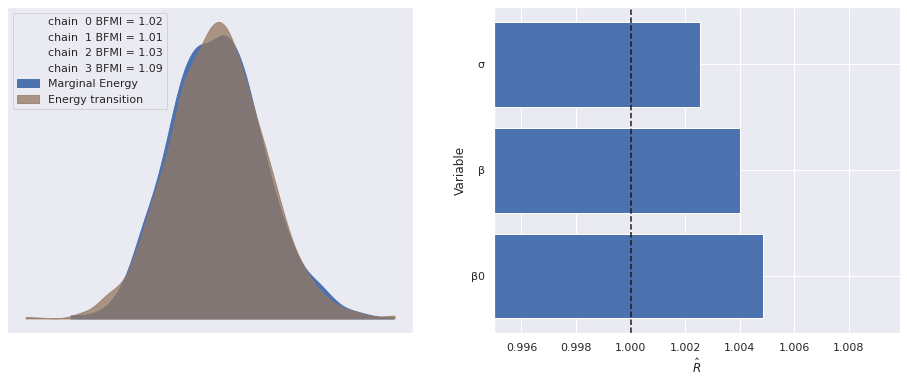The following plot shows excellent agreement between the actual and estimated values of the intercepts.

In :
ax, = az.plot_forest(multi_trace, var_names="β0", hdi_prob=0.95)

ax.scatter([], [],
facecolors='none', edgecolors='C0',
label="Posterior expected value");
ax.scatter(B0, ax.get_yticks()[::-1],
c='k', zorder=5,
label="Actual");

ax.set_yticklabels([]);
ax.set_ylabel(r"$\beta_0$");

ax.legend(loc="upper left");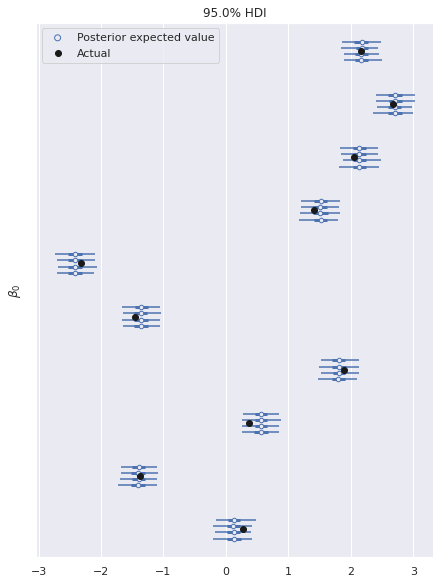There is similarly excellent agreement for the component coefficients.

In :
ax, = az.plot_forest(multi_trace, var_names="β",
coords={"β_dim_1": 0},
hdi_prob=0.95)

ax.scatter([], [],
facecolors='none', edgecolors='C0',
label="Posterior expected value");
ax.scatter(B[:, 0], ax.get_yticks()[::-1],
c='k', zorder=5,
label="Actual");

ax.set_yticklabels([]);
ax.set_ylabel(r"$\beta_{i, 0}$");

ax.legend(loc="upper left");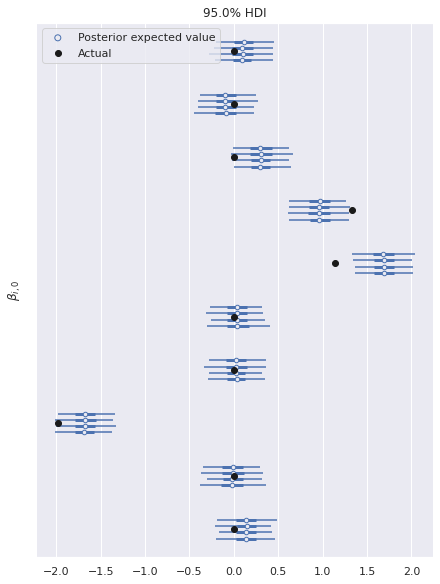Note that here we have only plotted the posterior distributions for the first column of $\beta$. The results are similar for the other columns.

In :
az.plot_posterior(multi_trace, var_names="σ", ref_val=S_SCALE);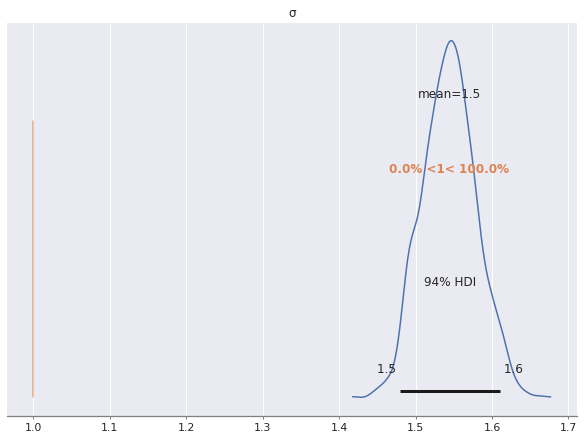As we can see, the posterior estimate of $\sigma$ is much higher than the true uncorrelated noise scale of one. This is due to the fact that we have not modeled the correlated noise induced by the latent factors. Indeed, since

$$\varepsilon_{i, j} = \mathbf{w}_j \cdot \mathbf{z}_i + \sigma_{i, j},$$

we get

\begin{align*} \operatorname{Var}(\varepsilon_{i, j}) & = \operatorname{Var}(\mathbf{w}_j \cdot \mathbf{z}_i + \sigma_{i, j}) \\ & = 2 \operatorname{Var}(w_{i, j}) \cdot \operatorname{Var}(z_{i, j}) + 1^2 \\ & = 3, \end{align*}

since $w_{i, j}$ and $z_{i, j}$ are independent standard normal variables, which are also independent of $\sigma_{i, j}$. Not accounting for the variation induced by the $\mathbf{w}_j \cdot \mathbf{z}_i$ term in this model has caused the estimate of the scale of $\sigma$ to be inflated.

### With latent factors¶

We now add latent factors to our model, starting with the most straightforward parametrization. The priors on $\beta_0$, $\beta$, and $\sigma$ are the same as for the previous model.

In :
with pm.Model() as factor_model:
β0 = pm.Normal("β0", 0., 5., shape=M)
β = pm.Normal("β", 0., 5., shape=(M, K))
μ = β0 + at.dot(X, β.T)

σ = pm.HalfNormal("σ", 2.5)


We place i.i.d. standard normal priors on the entries of $\mathbf{w}_j$ and $\mathbf{z}_i$. and add their product to the expected value of observations.

In :
with factor_model:
w = pm.Normal("w", 0., 1., shape=(M, F))
z = pm.Normal("z", 0., 1., shape=(N, F))

obs = pm.Normal("obs", μ + z.dot(w.T), σ, observed=Y)


Again we sample from the posterior distribution of this model.

In :
START_MULT = 3.

START_CORNERS = np.array([
[1., 1.],
[-1., 1.],
[-1., -1.],
[1., -1.]
])

w_starts = [
{"w": w_start, "z": np.ones((N, F))} for w_start
in START_MULT * np.ones((1, M, F)) * START_CORNERS[:, np.newaxis]
]

In :
with factor_model:
factor_trace = pm.sample(start=w_starts, **SAMPLE_KWARGS)

Auto-assigning NUTS sampler...
Multiprocess sampling (4 chains in 4 jobs)
NUTS: [β0, β, σ, w, z]

100.00% [8000/8000 03:11<00:00 Sampling 4 chains, 0 divergences]
Sampling 4 chains for 1_000 tune and 1_000 draw iterations (4_000 + 4_000 draws total) took 193 seconds.
The rhat statistic is larger than 1.4 for some parameters. The sampler did not converge.
The estimated number of effective samples is smaller than 200 for some parameters.


The $\hat{R}$ statistics for $\mathbf{z}_i$ and $\mathbf{w}_j$ are quite high and warrant investigation.

In :
make_diagnostic_plots(factor_trace);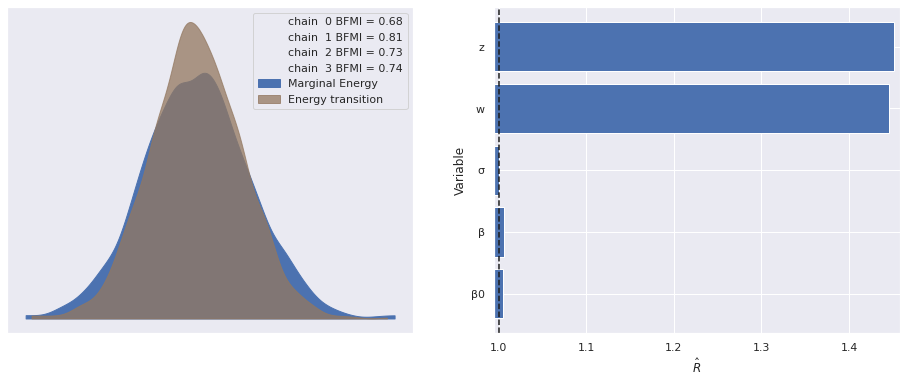In spite of the large $\hat{R}$ statistics, the estimate of the scale of $\sigma$ is quite accurate for this model, since we have accounted for the noise due to the latent variables.

In :
az.plot_posterior(factor_trace, var_names="σ", ref_val=S_SCALE);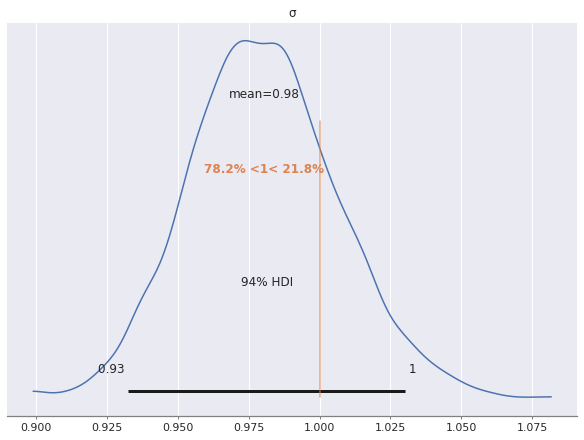We turn to the posterior distributions of the factor loadings $\mathbf{w}_j$. Below we plot the first two entries in $\mathbf{w}_0$ and $\mathbf{w}_1$ against each other.

In :
ax = az.plot_pair(factor_trace, var_names="w",
coords={"w_dim_0": [0, 1], "w_dim_1": 0},
scatter_kwargs={'alpha': 0.5})

ax.set_xlabel("$w_{0, 0}$");
ax.set_ylabel("$w_{1, 0}$");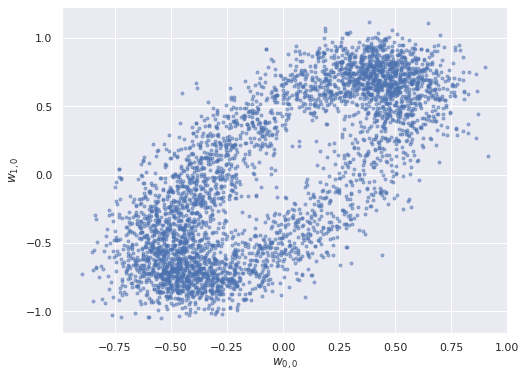The elliptical shape of this posterior distribution is certainly unusual; let's see what the pairwise plots look like for all pairs of entries in the factor loadings.

In :
axes = az.plot_pair(factor_trace, var_names="w",
scatter_kwargs={'alpha': 0.5})

for ax in axes.flat:
ax.set_xlabel(None);
ax.set_ylabel(None);

axes[0, 0].figure.tight_layout();